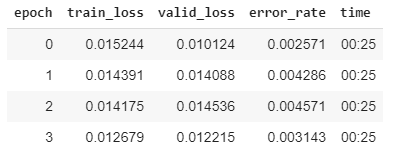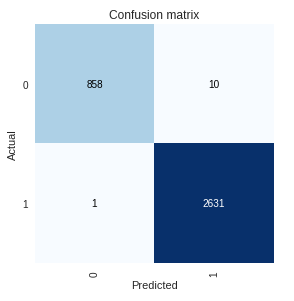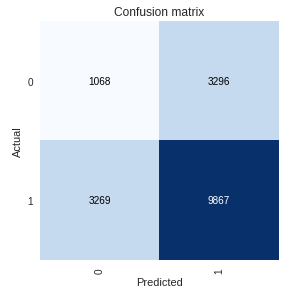# Very poor prediction results on whole training set

I am facing a weird problem which I am not being able to explain. I have an image dataset which I am training on. Training results are quite good and do not seem to be overfitting as validation loss is comparable to training loss.

``````data = ImageDataBunch.from_csv(path_train, csv_labels=path/'train.csv', ds_tfms=get_transforms(), size=32, bs=128
).normalize(imagenet_stats)
learn = cnn_learner(data, models.resnet34, metrics=error_rate)
``````The confusion matrix is also quite good.Now when I am trying to make predictions for the whole training set, my results are pathetic which is really bizarre because this is the same dataset on which I trained my near accurate model.

preds_train, y_train, losses_train = learn_pred.get_preds(ds_type=DatasetType.Test, with_loss=True)

The ROC area under curve is under 0.5 and the confusion matrix is very poor.Next I tried normalizing the data before calling`get_preds()` with the same results.

tfms=get_transforms()
test_data = (ImageList.from_folder(path_train)
.split_none()
.label_empty()
.transform(tfms, size=32)
.databunch()
.normalize(imagenet_stats))

I even called `predict()` for each individual image and got the same results.

``````pred = []
for img in test_data.train_ds.x:
pred.append(learn_pred.predict(img))
``````

I am failing to figure out what I am doing wrong. Would really appreciate if someone could guide me.

I am not sure and might be wrong, but aren’t you supposed to make predictions on completely new data, something your model has not seen yet?

yes, that’s correct. But shouldn’t I be able to make predictions on the training set as well? For example, scikit-learn allows you to do that. Because the model knows the training data, the results are quite good and that is expected, however, here it’s acting quite the opposite and giving me poor results on data it has seen before!